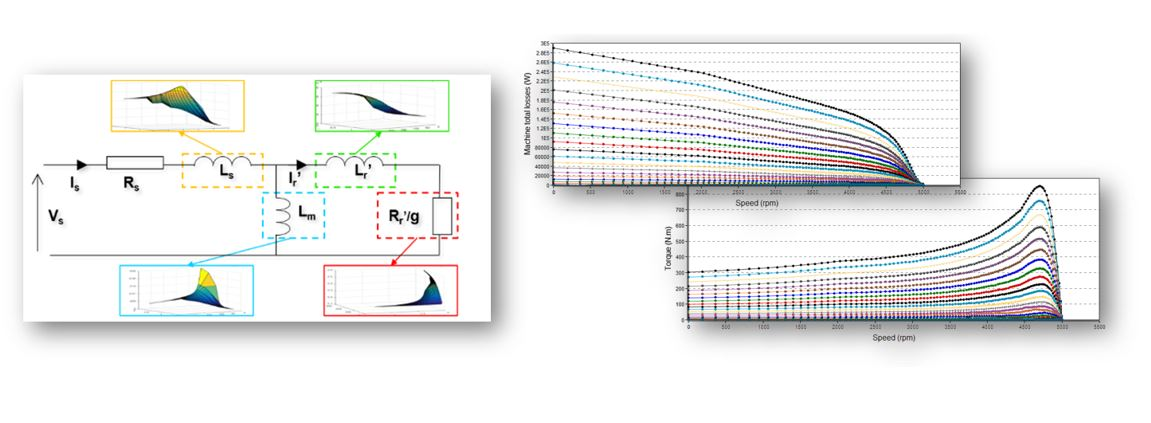# Char. - Model - Motor - Scalar map

## Positioning and objective

The aim of the test “Characterization – Model – Motor – Scalar” is to characterize the behavior of the machine in function of a set of Line-Line voltage (U) and a set of power supply frequency (f) (only motor operating mode available). Those computations are based on the identification and the solving of a non-linear model considering the cross saturation and the eddy current effects. All the main electromagnetic quantities are computed and displayed as curves in function of speed for a given power supply frequency and a set of Line-Line voltages.“Characterization – Model – Motor – Scalar” Illustration

The results of this test give an overview of the electromagnetic behavior of the machine considering its topology. For a set of Line-Line voltages (U) and a set of power supply frequencies (f), the general parameters of the machine like, mechanical torque, currents, power factor and power balance are computed and displayed as curves.

This gives the capability to make comparisons between the results obtained from the measurements and those with the Altair® FluxMotor®.

In this test, system engineers will find a characterization tool adapted to their needs and able to provide accurate curves ready to be used in system simulation software like, Activate or PSIM. Indeed, from results obtained in this test, a scalar drive and control can be applied.

Note: We consider the motor convention.

The following table classifies the test “Characterization – Model – Motor – Scalar

 Family Characterization Package Model Convention Motor Test Scalar Positioning of the test "Characterization - Model - Motor - Scalar

## User input

The four main user input parameters are the maximum Line-Line voltage, the Rated power supply frequency, the maximum line current and the maximum speed. In addition, the temperatures of winding and squirrel cage must be set in the power electronics, if needed.

## Main outputs

Curves versus speed for a set of Line-Line voltage (U) and a set of power supply frequency (f)
1. Mechanical torque
2. Mechanical power
3. Machine efficiency
4. System efficiency
5. Machine electrical power
6. System electrical power
7. Machine total losses
8. System total losses
9. Stator total losses
10. Rotor total losses
11. Mechanical total losses
12. Power electronics losses
13. Power factor
14. Stator current